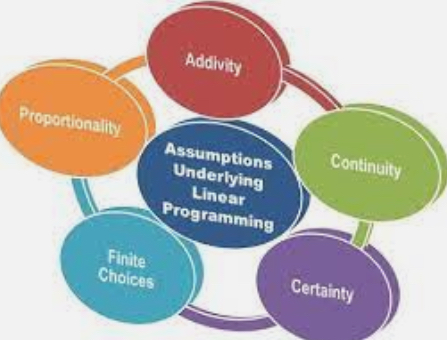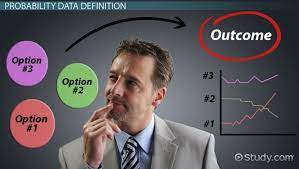Linear programming is an optimization technique for a system of linear constraints and a linear objective function. An objective function defines the quantity to be optimized, and the goal of linear programming is to find the values of the variables that maximize or minimize the objective function.
The resources in the organization are usually scarce and limited in supply in order to achieve the objectives of the business
Thus, managers may have to use tools and techniques to enable them optimize and manage their limited resources
The tool that helps managers optimize their limited resources is known as Linear Programming. Linear programming (LP) is an important technique for optimization of resources. The word Linear means the rela¬tionships between different variables
The word Programming means planning it also refers to the process of selecting best course of action from various alterna¬tives.
Thus, linear programming is a mathematical technique for allocating limited resources in an optimal manner
A linear programming problem is one that is concerned with finding the optimal value (maximum or minimum) of a linear function of several variables (called objective function) subject to the conditions that the variables are non-negative and satisfy a set of linear inequalities (called linear constraints)

Objective
The linear programming technique is used for selecting the best possible strategy from a number of alternatives. Therefore, the main objective of Linear Programming is to optimize our objectives given a set of constraints.

Applications
• LP technique is applied to a wide variety of problems:
• Maximize profit with optimal production processes
• Minimize cost while meeting product specifications
• Optimizing the product mix when the production line works under certain specification
• Minimize cost in transportation routes
• Utilizing the storage and distribution centres
• Minimizing the raw materials
• Find cheapest combination of food that will satisfy nutritional requirements
• LP can be applicable in various fields such as Operations, Accounting, Marketing, Banking, Agriculture, Logistics, Engineering, Medicine.

Characteristics of Linear Programming
• All linear programming problems must have following five characteristics:
• Objective function
• Constraints
• Non-negativity
• Linearity
• Finite

Assumptions of Linear Programming
• Resources are limited
• There is only one objective
• The objective function is to be optimized that is profit maximization or cost minimization.
• The relationship between objective function and constraints are linear.
• There are a number of constraints or restrictions- expressible in quantitative terms
• Data are available to specify the problem
• Non-negativity constraints

Methods of Solving Linear Programming
Graphical Method
The business problems involving only two variables can be solved by drawing the graph for various constraints
Simplex Method
The business problems involving two or more variables can be easily solved using Simplex Method.
The simplex method is an algorithm for solving more complex problems.

Illustration
A factory manufactures doodads and whirligigs. It costs \$2 and takes 3 hours to produce a doodad. It costs \$4 and takes 2 hours to produce a whirligig. The factory has \$220 and 150 hours this week to produce these products. If each doodad sells for \$6 and each whirligig sells for \$7, then how many of each product should be manufactured this week in order to maximize profit?

This kind of problem is perfect to use linear programming techniques on.
All of the quantifiable relationships in the problem are linear.
The values of variables are constrained in some way.
The goal is to find values of the variables that will maximize some quantity.

# MMBA3## PROBABILITY – A TOOL FOR DECISION MAKING

in## “MAXIMIZING BALANCE SHEET: UNLOCKING THE PATH TO ORGANIC GROWTH AND FINANCIAL SUCCESS.

in